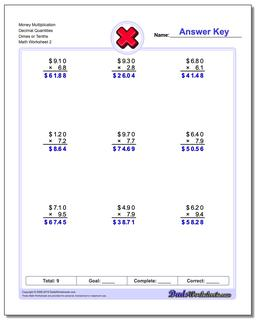Math Worksheets

### Worksheet News

PLEASE GO BACK AND USE THE BIG BLUE 'PRINT' BUTTON ON THE PAGE TO PRINT THE WORKSHEET CORRECTLY!Sorry for the trouble! The browser won't print the embedded worksheet PDF directly using the normal 'Print' command in the file menu, so you need to click the big 'Print' button to send just the worksheet and not the surrounding page to the printer.

# Math Worksheets: Multiplication: Multiplication: Money Multiplication Decimal Quantities Dimes or Tenths (Second Worksheet)## Money Multiplication Decimal Quantities Dimes or Tenths (Second Worksheet)

Property Value
DescriptionMoney Multiplication Decimal Quantities Dimes or Tenths: Multiplication worksheets that multiply dollars times whole and decimal unit quantities. Some results will require rounding. (Second Worksheet)
Resource TypeWorksheet